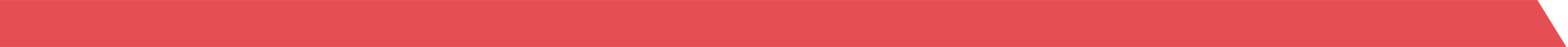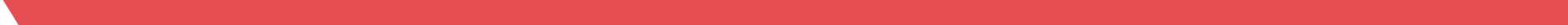## TipsFix# How to Convert Fractional Odds to Decimal 24 February 2021

If you’re new to online betting, you may have noticed that odds are sometimes displayed differently on different bookmakers’ websites. The majority of bookmakers such as the ones found on telecomasia.net display odds as fractions which is what most UK punters are used to. However, some display decimal odds which can be confusing to some if you haven’t used them before and so we’ve put together a quick guide to show you how you can easily convert fractional odds into decimal odds and vice versa.

It’s worth noting that you can almost always change the format of the odds on a bookmakers website and so you shouldn’t have to convert them manually. This can usually be done with the click of a button somewhere in the settings. This guide is to help you understand how the different formats of odds work and how you can change them if need be.

## Fractional Odds

As mentioned above, fractional odds are most commonly used with UK bookmakers and you’ll often see them on bookie advertisements on their website or on TV.

Some examples of fractional odds are:

• 2/1
• 5/1
• 7/2
• 13/2

Fractional odds are relatively simple to understand. In order to win the number on the left, you must stake the number on the right. Note that the number on the left will be the profit you make and not your overall returns. Your returns will also include your stake.

For example, if the odds were 4/1, if you staked £1 on a bet, you would make £4 profit (£5 total return).

If the odds were 13/2, if your stake on a bet was £2, the profit you would make if it won would be £13 (£15 total return).

The slight issue with fractional odds is when your stake is not, or not a multiple of, the number on the right.

For example, if the odds were 13/2 and you wanted to stake £3.75, it becomes a little trickier to work out your profit and returns.

## Decimal Odds

Decimal odds are most commonly used on online betting exchanges. In a way, they’re much easier to use than fractional odds.

Some examples of decimal odds are:

• 2.0
• 3.15
• 1.57
• 12.50

To calculate your returns from a bet using decimal odds, you simply multiply your stake by the odds. Note that this number will give you your total returns (including stake) and not your just profit.

For example, if the decimal odds were 2.0 and you bet £10, if your bet won, your total returns would be £20 (£10 profit).

If the odds were 8.50 and your stake was £10, your returns would be £85 (£75 profit).

## Converting Fractional Odds To Decimal

If you need to convert fractional odds into decimal odds, there is a simple way to do it.

You simply need to divide the first number by the second number in the fractional odds and then add 1.

For example, if the fractional odds were 2/1, you would divide 2 by 1 (2) and add 1 to give a total of 3.0 which is the decimal version of 2/1.

If the odds were 13/2, you would divide 13 by 2 (6.5) and add 1 to give 7.50.

There are multiple odds conversion tools online which can help you convert fractional odds into decimal and vice versa as well as free apps in the app stores.

Remember that if you’re using a bookmakers website, there will be an option to automatically change the format of the odds. If you can’t fid the setting for it, simply contact support who will advise you on how to change them.